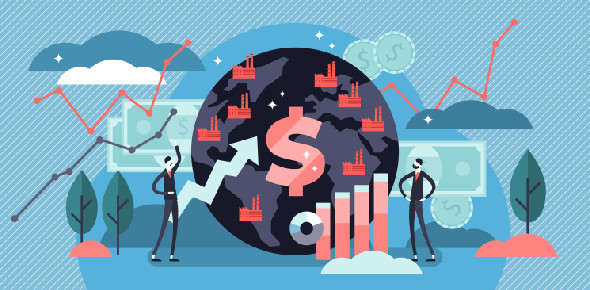# Macroeconomics [ch. 21]

35 Questions | Total Attempts: 823SettingsThe influence of monetary and fiscal policy on aggregate demand

Related Topics
• 1.
An increase in the interest rate increases the quantity demanded of money because it increases the rate of return on money
• A.

True

• B.

False

• 2.
• A.

True

• B.

False

• 3.
Keynes's theory of liquidity preference suggests that the interest rate is determined by the supply and demand for money
• A.

True

• B.

False

• 4.
The interest-rate effect suggests that aggregate demand slopes downward because an increase in the price level shifts money demand to the right, increases the interest rate, and reduces investment
• A.

True

• B.

False

• 5.
An increase in the money supply shifts the money supply curve to the right, increases the interest rate, decreases investment, and shifts the aggregate-demand curve to the left
• A.

True

• B.

False

• 6.
Suppose investors and consumers become pessimistic about the future and cut back on expenditures.  If the Fed engages in activist stabilization policy, the policy response should be to decrease the money supply
• A.

True

• B.

False

• 7.
In the short run, a decision by the Fed to increase the money supply is essentially the same as a decision to decrease the interest rate target
• A.

True

• B.

False

• 8.
Because of the multiplier effect, an increase in government spending of \$40 billion will shift the aggregate-demand curve to the right by more than \$40 billion (assuming there is no crowding out)
• A.

True

• B.

False

• 9.
If the MPC (marginal propensity to consume) is .80, then the value of the multiplier is 8
• A.

True

• B.

False

• 10.
Crowding out occurs when an increase in government spending increases incomes, shifts money demand to the right, raises the interest rate, and reduces private investment
• A.

True

• B.

False

• 11.
Suppose the government increases its expenditure by \$10 billion.  If the crowding-out effect exceeds the multiplier effect, then the aggregate-demand curve shifts to the right by more than \$10 billion
• A.

True

• B.

False

• 12.
Suppose investors and consumers become pessimistic about the future and cut back on expenditures.  If fiscal policymakers engage in activist stabilization policy, the policy response should be the decrease government spending and increase taxes
• A.

True

• B.

False

• 13.
Many economists prefer automatic stabilizers because they affect the economy with a shorter lag than activist stabilization policies
• A.

True

• B.

False

• 14.
In the short run, the interest rate is determined by the loanable-funds market, while in the long run, the interest rate is determined by money demand and money supply
• A.

True

• B.

False

• 15.
Unemployment benefits are an example of an automatic stabilizer because when incomes fall, unemployment benefits rise
• A.

True

• B.

False

• 16.
Keynes's liquidity preference theory of the interest rate suggests that the interest rate is determined by
• A.

They supply and demand for loanable funds

• B.

The supply and demand for money

• C.

The supply and demand for labor

• D.

Aggregate supply and aggregate demand

• 17.
• A.

Increases the quantity demanded of money

• B.

Increases the demand for money

• C.

Decreases the quantity demanded of money

• D.

Decreases the demand for money

• E.

Does none of the above

• 18.
When the supply and demand for money are expressed in a graph with the interest rate on the vertical axis and the quantity of money on the horizontal axis, an increase in the price level
• A.

Shifts money demand to the right and increases the interest rate

• B.

Shifts money demand to the left and increases the interest rate

• C.

Shifts money demand to the right and decreases the interest rate

• D.

Shifts money demand to the left and decreases the interest rate

• E.

Does none of the above

• 19.
For the United States, the most important source of the downward slope of the aggregate-demand curve is
• A.

The exchange-rate effect

• B.

The wealth effect

• C.

The fiscal effect

• D.

The interest-rate effect

• E.

None of the above

• 20.
In the market for real output, the initial effect of an increase in the money supply is to
• A.

Shift aggregate demand to the right

• B.

Shift aggregate demand to the left

• C.

Shift aggregate supply to the right

• D.

Shift aggregate supply to the left

• 21.
The initial effect of an increase in the money supply is to
• A.

Increase the price level

• B.

Decrease the price level

• C.

Increase the interest rate

• D.

Decrease the interest rate

• 22.
The long-run effect of an increase in the money supply is to
• A.

Increase the price level

• B.

Decrease the price level

• C.

Increase the interest rate

• D.

Decrease the interest rate

• 23.
Suppose a wave of investor and consumer pessimism causes a reduction in spending. If the Federal Reserve chooses to engage in activist stabilization policy, it should
• A.

Increase government spending and decrease taxes

• B.

Decrease government spending and increase taxes

• C.

Increase the money supply and decrease interest rates

• D.

Decrease the money supply and increase interest rates

• 24.
The initial impact of an increase in government spending is to shift
• A.

Aggregate supply to the right

• B.

Aggregate supply to the left

• C.

Aggregate demand to the right

• D.

Aggregate demand to the left

• 25.
If the marginal propensity to consume (MPC) is .75, the value of the multiplier is
• A.

.75

• B.

4

• C.

7.5

• D.

None of the above